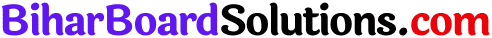# Bihar Board 12th Economics Objective Answers Chapter 1 Introduction to Micro Economics

Bihar Board 12th Economics Objective Questions and Answers

## Bihar Board 12th Economics Objective Answers Chapter 1 Introduction to Micro Economics

Question 1.
Which Economist divided Economics in two branches of micro and macro on the basis of economic activity ?
(A) Marshall
(B) Ricardo
(C) RagnarFrish
(D) None of these
(D) None of theseQuestion 2.
Which of the following is studied under Micro Economics ?
(A) Individual unit
(B) Economic Aggregate
(C) National Income
(D) None of these
(D) None of these

Question 3.
Which of the following economic activities are included in the subject-matter of Economics?
(A) Economic Activities related to Unlimited Wants
(B) Economic Activities related to Limited Resources
(C) Both (a) and (b)
(D) None of these
(C) Both (a) and (b)

Question 4.
On which base structure of economic problems has been installed?
(A) Unlimited Wants
(B) Limited Resources
(C) Both (a) and (b)
(D) None of the above
(C) Both (a) and (b)

Question 5.
‘Micros’, which means ‘Small’ belongs to:
(A) Arabian word
(B) Greek word
(C) German word
(D) English word
(B) Greek wordQuestion 6.
Which of the following statement is true?
(A) Human wants are infinite
(B) Resources are limited
(C) Scarcity problem gives birth to choice .
(D) All of these
(C) Scarcity problem gives birth to choice

Question 7.
Which of the following is the salient feature of factors (or resources) ?
(A) These are limited as compared to wants
(B) These have alternative uses
(C) Both (a) and (b)
(D) None of the above
(C) Both (a) and (b)

Question 8.
Which is a central problem of an economy ?
(A) Allocation of Resources
(B) Optimum Utilisation of Resources
(C) Economic Development
(D) All of these
(D) All of theseQuestion 9.
Which of the following Is a type of economic activities ?
(A) Production
(B) Consumption
(C) Exchange and Investment
(D) All of these
(D) All of these

Question 10.
To which factor, economic problem is basically related to:
(A) Choice
(B) Consumer’s Selection
(C) Firm Selection
(D) None of these
(A) Choice

Question 11.
Economy may be classified as:
(A) Capitalist
(B) Socialist
(C) Mixed
(D) All of these
(D) All of theseQuestion 12.
Which economy has a co-existence of private and public sectors ?
(A) Capitalist
(B) Socialist
(C) Mixed
(D) None of these
(C) Mixed

Question 13.
The main objective of a socialist economy is t
(A) Maximum production
(B) Economic freedom
(C) Earning profit
(D) Maximum public welfare
(D) Maximum public welfare

Question 14.
In which economy decisions are taken on the basis of price mechanism ?
(A) Socialist
(B) Capitalist
(C) Mixed
(D) All of these
(B) CapitalistQuestion 15.
The slope of a production possibility curve falls:
(A) From left to right
(B) From right to left
(C) From top to bottom
(D) From bottom to top
(C) From top to bottom

Question 16.
Production Possibility Curve is:
(A) Concave to the axis
(B) Convex to the axis
(C) Parallel to the axis
(D) Vertical to the axis
(A) Concave to the axis

Question 17.
Mention the name of the curve which shows economic problem:
(A) Production Curve
(B) Demand Curve
(C) Indifference Curve
(D) Production Possibility Curve
(D) Production Possibility CurveQuestion 18.
Which of the following is studied under Macro Economics ?
(A) National Income
(B) Full. Employment
(C) Total Production
(D) All of these
(D) All of these

Question 19.
Which of the following Is a branch of Micro Economics ?
(A) Product Price Determination
(B) Factor Price Determination
(C) Economic Welfare
(D) All of these
(D) All of these

Question 20.
Which of the following is a source of production ?
(A) Land
(B) Labour
(C) Capital
(D) All of these
(D) All of these

Question 21.
Who said, “Economics is a science of wealth.”
(A) Marshall
(B) Robbins
(D) J.K. MehtaQuestion 22.
“Economics is a science of logic.’’ Who said it ?
(A) Hicks
(B) Keynes
(C) Robbins
(D) Marshall
(C) Robbins

Question 23.
Micro Economics includes:
(A) Individual unit
(B) Small units
(C) Individual price determination
(D) All of these
(D) All of these

Question 24.
Who was the father of Economics ?
(A) I. B. Say
(B) Malthus
(D) Joan Robinson

Question 25.
Who gave the definition of Economics related to welfare ?
(B) Marshall
(C) Robbins
(D) Samuelson
(B) MarshallQuestion 26.
The word ‘micro’ was firstly used by:
(A) Marshall
(B) Boulding
(C) Keynes
(D) Ragnar Frish
(D) Ragnar Frish

Question 27.
According to whom, Economics is a sciencee of human welfare ?
(A) A Marshall
(B) Paul Samuelson
(C) J. S. Mill
(A) A Marshall

Question 28.
Which of the following is not a factor of production ?
(A) Land
(B) Labour
(C) Money
(D) Capital
(C) Money

Question 29.
The central problem of an economy is:
(A) What to produce ?
(B) How to produce ?
(C) How to distribute produced goods ?
(D) All of these